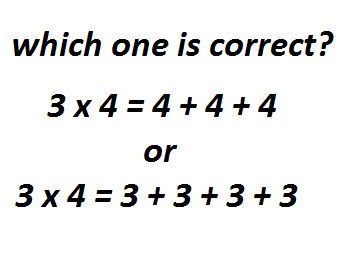in STEMGeeks5 days agoLearning mathematics is a necessity that we cannot avoid. Mathematics guides us to be more careful in this life. That's why we need to teach mathematics to our children well.
In mathematics we will learn to count starting from addition, subtraction, division and multiplication. This time I want to write about multiplication in mathematics. When we were in 2nd grade, we started learning multiplication. Multiplication is defined as repeated addition.
However, in mathematical and real life concepts, the number that is repeated is the number behind.
As an example

### = 12

Let's look at the multiplication above. The repetition that is done is number 4 three times. What we have to pay attention to is the number that is repeated is the number that is behind.

## Commutative property of expression

As we get older and mature we can count in other ways. Repeatedly adding the number 3 four times. It turns out that we also get the same result which is 12. This is all because we are also taught at school that multiplication has a commutative property. This means that the multiplication of the numbers being multiplied can change places.
Let's take another look at the example above

### 3 x 4

With the commutative property, the multiplication can be written as

It means,

### 3 X 4 = 4 x 3.

With a commutative nature, the number 3 in front of it can swap places with the number 4. As a result, in the practice of calculating multiplication we also often repeat the number 3 4 times. In this repetition the result remains the same, namely 12.

## Logic of prescription drugs from doctors

With a different calculation method but the result is the same, the question arises which calculation is correct?
To answer this question, we need to look at logic in our daily lives. The closest thing is to see the rules for taking medication from the doctor. We often find on prescription drugs that patients must consume.

### 3 x 1

The rule states that patients must take the drug three times with a single dose. It could be in the morning one dose/tablet or capsule, in the afternoon one dose/tablet or capsule, and in the evening one dose/tablet/capsule.
The rules for taking medicine are not written

### 1 x 3

Which means that you drink three doses/tablets or capsules at once.
Well, from here we can see there is a difference. In the rule of drinking 3 x 1 is not the same as 1 x 3 even though in the count the results are the same. Because, if the patient takes three doses of the drug at once, it can endanger the patient himself.
By looking at the logic of taking this medicine, we can see that multiplication is repeated addition with numbers behind.

### = 27

So we can explain to the children about multiplication correctly.
Thus all my writing this time may useful for all of us.

Best regard from Indonesia.
@rokhani

# Thank you for stopping by and reading my post. I hope you enjoy it. Upvote, comment, reblog and other support are highly appreciated.

Posted with STEMGeeks

Sort:

ဗဟုသုတရပါတယ်

5 days ago Reveal Comment